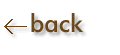Steps in Graphing:

1)  Determine the scale to be used for the x-axis:  determine the smallest and largest values to be graphed, find the difference, then divide by the approximate number of spaces available on the graph paper.  Use a convenient spacing.  Mark off the x-axis beginning with the smallest number at the origin (not necessarily zero).

2)  Repeat the same process for the y-axis.

3)  Clearly label the axes.  Indicate the variable represented and the unit of measurement.

4)  Label the graph as a whole.

5)  Plot the points.

6)  Draw a smooth curve (not a jagged line) which will average out experimental error.